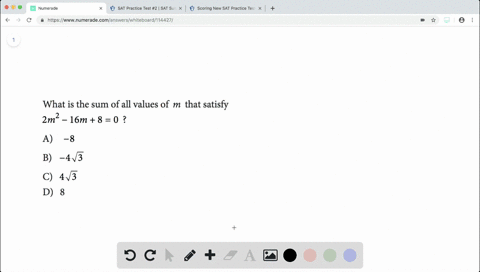Enroll in one of our FREE online STEM summer camps. Space is limited so join now!View Summer Courses### What is the sum of all values of $m$ that satisfy…

02:40Johns Hopkins University

Need more help? Fill out this quick form to get professional live tutoring.

Get live tutoring
Problem 12

Over which of the following time periods is the
average growth rate of the sunflower least?
$$\begin{array}{l}{\text { A) } \operatorname{Day} 0 \text { to Day } 21} \\ {\text { B) Day } 21 \text { to Day } 42} \\ {\text { C) Day } 42 \text { to Day } 63} \\ {\text { D) Day } 63 \text { to Day } 84}\end{array}$$

Check back soon!
SAT
SAT Practice Test # 6

## Discussion

You must be signed in to discuss.

## Video Transcript

So for this one we're looking for when the average growth rate is the least And so we're looking for one that rate is the lease. So those are key words there? Well, we know that and word problems that in terms of our equation if we had, like, a Y is equal to M. Iss was feverish. This isn't an X m s plus B, but if we were to split it up, it would roughly be you. And I'm explodes. Be many m explosive. He's basically okay. So then for this, we know that M is representative of our slow. So we always know this stuff is our rate. So then if we know that way, it's so bizarre rate and we want to know what the rate is the least That means when the slope is the smallest. Okay, so if you're looking for when the slope is the smallest, we could do like it here and clearly in the beginning, it's increasing very by a large amount of o tio. I expect this craft a little more extremes like this. So at the beginning and in the middle, it's going really fast. And then at the end. It's kind of tapering off. So at the very end between days sixty three eighty for the slope is gonna be the least to the rate is the least.